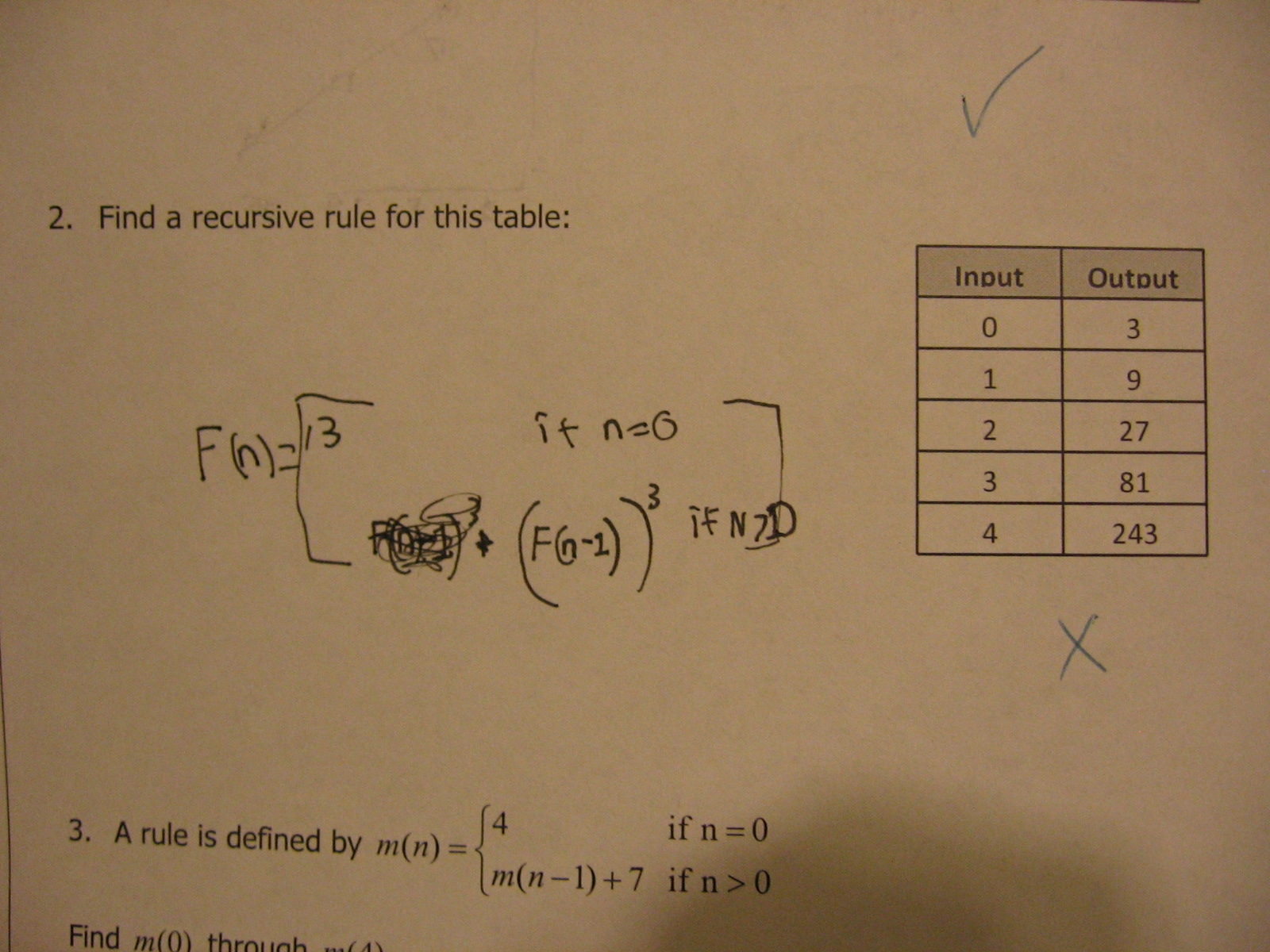Categories

# Matching a recursive function to a tableWhat does the student know? What’s the mistake? How would you help?

## 2 replies on “Matching a recursive function to a table”

tieandjeanssays:

They know to scan the page for clues about how to proceed, which gives the piecewise 0-expection. They know that a recursive rule is one that defines the nth terms in terms of n’s neighbors.

But the confusion between a function that uses N+1 or N-1 as a value and something that’s recursive is missing. Even the scratched out answer shows this confusion. f(n) = (n+1)^3 is a good rule for the table, but it’s not recursive. Right? I mean, I could be embarrassing myself here. My expectation for a recursive rule would be something of the form

F(n) = value + f(n-1)

where there’s a clear base case value defined.

I’m curious what the “correct” form of this answer looks like. Is there a specific format that students have been trained in?

In programming classes, I do my best to avoid teaching recursion “just ’cause.” Since this has an obvious non-recursive expression, it makes recursion seem like another arrow in the quiver of math sadism, instead of a profound and useful tool for expressing complex solutions.

mathisfun...damental!says:

Given the table above, the recursive formula should be f(n)=3f(n-1) where f(0)=3. To help a student figure it out, I would suggest they think of just the numbers in the output column and how they relate to the number before it. Students often get confused because they try to find a relationship between the input and the output variables, but in a recursive formula, it’s easiest to only focus on the output column to find your pattern.

Hope this helps. I know your post was from a long time ago, so you probably have already figured this out. 🙂